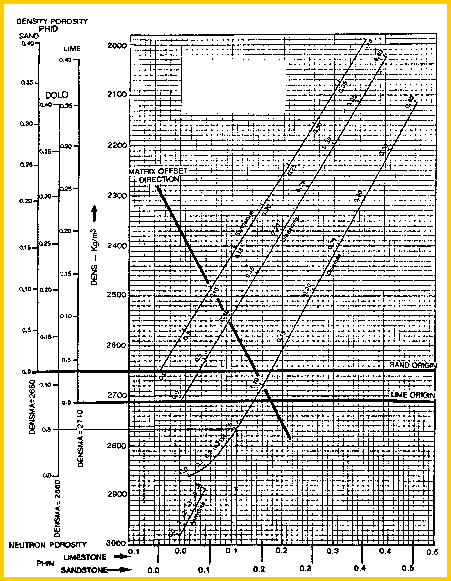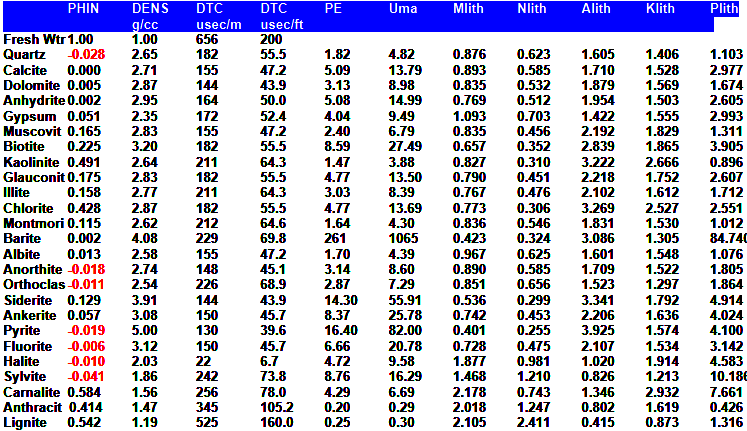Lithology from Matrix Density
The response equation for the density log follows the classical form:
1: DENS = PHIe * Sxo * DENSw (water term)
+ PHIe * (1 - Sxo) * DENSh (hydrocarbon term)
+ Vsh * DENSsh (shale term)
+ (1 - Vsh - PHIe) * Sum (Vi * DENSi) (matrix term)

Where:
DENSh = log reading in 100% hydrocarbon
DENSi = log reading in 100% of the ith component of matrix rock
DENSHsh = log reading in 100% shale
DENSw = log reading in 100% water
PHIe = effective porosity (fractional)
Sxo = water saturation in invaded zone (fractional)
Vi = volume of ith component of matrix rock
Vsh = volume of shale (fractional)

By rearranging the density response equation, we can derive apparent matrix density, based on the final effective porosity, shale volume, and the density log reading. Sxo is assumed to be 1.0. The matrix density thus derived can be compared to the known matrix density data to find an approximate lithology.

Calculate density log value from porosity log reading.
2: DENS = PHID * KD1 + (1 - PHID) * KD2
3: DENSSH = PHIDSH * KD1 + (1 - PHIDSH) * KD2
Calculate matrix density
4: IF Vsh + PHIe < 0.95
5: THEN DENSma = (DENS - PHIe * DENSW - Vsh * DENSSH) / (1 - PHIe - Vsh)
6: OTHERWISE DENSma = DENS
Calculate mineral volumes (relative to each other)
7: Vmin1 = (DENSma - DENS2) / (DENS1 - DENS2)
8: Vmin2 = 1 - Vmin1
Calculate mineral volumes (relative to total rock volume)
9: Vrock = (1.0 - Vsh - PHIe)
10: V1 = Vmin1 * Vrock
11: V2 = Vmin2 * Vrock

Where:
DENS = density log reading (gm/cc or kg/m3)
DENS1 = density of first mineral (gm/cc or kg/m3)
DENS2 = density of second mineral (gm/cc or kg/m3)
DENSma = calculated matrix density (gm/cc or kg/m3)
DENSSH = density log reading in shale (gm/cc or kg/m3)
DENSW = density log reading in water (gm/cc or kg/m3)
PHID = porosity log reading (fractional)
PHIe = effective porosity from any method (fractional)
PHIDSH = porosity log reading in shale (fractional)
Vsh = volume of shale (fractional)
Vrock = rock volume (fractional)
Vmin1 = volume of first mineral (fractional)
Vmin2 = volume of second mineral (fractional)

Volumes for lithology track:
V1 = relative volume of first mineral (fractional)
V2 = relative volume of second mineral (fractional)Constants
English Units   Metric Units    Log Scale
KD1       1.00                 1000               All
KD2       2.65                 2650           Sandstone
KD2       2.71                 2710           Limestone
KD2       2.87                 2870           DolomiteCOMMENTS:
This equation will break down when PHIe plus Vsh approaches 1.0, so we limit the use of the equation to those cases where PHIe + Vsh < 0.95.

The matrix density will be too low in gas zones and in rough hole. No obvious correction can be made to overcome this problem. Do not use apparent matrix densities under these conditions.

The standard density neutron crossplot can be used to determine matrix density, as shown below.Density neutron crossplot to find DENSmaNUMERICAL EXAMPLE:
1. For Sand D in Example 1:
PHID = 0.12
PHIe = 0.11
Vsh = 0.33
DENSW = 1000 kg/m3
DENSMA = 2150 kg/m3
DENS = 0.12 * 1.00+ (1 - 0.12) * 2.65 = 2.452
DENSSH = 0.03 * 1.00 + (1 - 0.03) * 2.65 = 2.600
DENSW = 1.000
DENSma = (2.452 - 0.11 * 1.0 - 0.33 * 2.65) / (1 - 0.11 - 0.33) = 2.620 g/cc

2. If we use the Vsh from the density neutron crossplot (Vsh = 0.59)
DENSma = (2.452 - 0.11 * 1.0 - 0.59 * 2.65) / (1 - 0.11 - 0.59) = 4.680 g/cc

This is an impossible result which suggests that the shale volume or the effective porosity is wrong. Calculated matrix density can thus be used as a quality control indicator when it exceeds reasonable bounds.

3. Assume the two mineral model consists of quartz and dolomite and the computed matrix density is 2680 kg/m3. Then:
Vsh = 0.10
PHIe = 0.20
DENS1 = 2650 kg/m3 (Sandstone)
DENS2 = 2870 kg/m3 (Dolomite)

Vrock = 1 - 0.10 - 0.20 = 0.70
Vmin1 = (2680 - 2870) / (2650 - 2870) = 0.86
Vmin2 = 1.00 - 0.86 = 0.14

Volumes for lithology track:
V1 = 0.86 * 0.70 = 0.60
V2 = 0.14 * 0.70 = 0.10

PHIe + Vsh + Vmin1 + Vmin2 = 0.20 + 0.10 + 0.60 + 0.10 = 1.00

This rock is composed of 10% shale, 20% porosity, 60% quartz, 10% dolomite. The quartz/dolomite ratio is 0.86 to 0.14.= 6.14.DENSITY LITHOLOGY CODES
To produce a lithology code on computer listings, we bracket the DENSma values as follows:

DlithCode - Density Lithology Codes

DENSma                              DlithCode
< 2630 and bad hole              HOLE
< 2630 and coal trigger set    COAL
< 2630 and good hole            GAS
2630 - 2659                             QRTZ
** 2660 - 2699                         LMSD
** 2700 - 2729                         LIME
** 2730 - 2799                         LMDL
2800 - 2879                            DOLO
2880 - 3149                            ANHY
3150 and above                    HEVY
no value computed                 ----
any value and Vsh > 0.85      SHLE
** Could be DLSD if PE < 3.0

Evaporites require special handling in computer programs and the above codes should be expanded to include:
DENSma                               DLITHCODE
2500 - 2629                        gas or bad hole as above
2300 - 2499                              GYPS
2000 - 2299 and low sonic       SALT
2000 - 2299 and high sonic      SULF
1800 - 1999                              SYLV
1500 - 1799                              CARNPARAMETERSPage Views ---- Since 01 Jan 2015
Copyright 2023 by Accessible Petrophysics Ltd.
CPH Logo, "CPH", "CPH Gold Member", "CPH Platinum Member", "Crain's Rules", "Meta/Log", "Computer-Ready-Math", "Petro/Fusion Scripts" are Trademarks of the Author